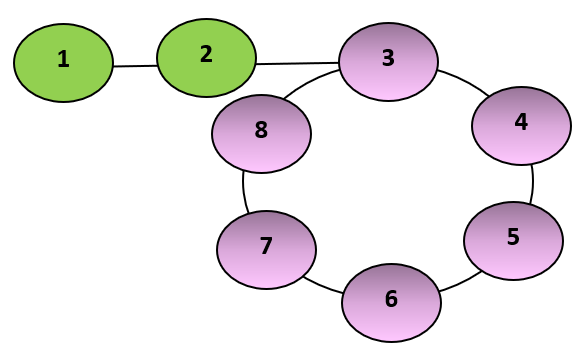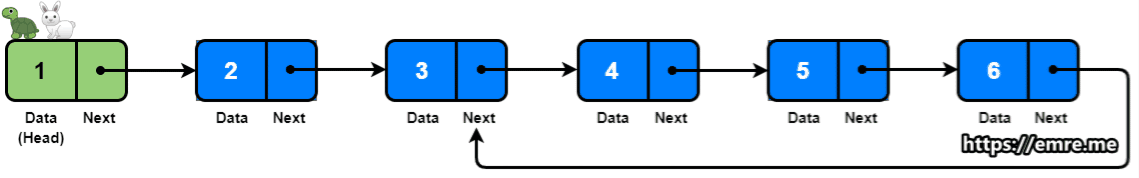# Length of the loop in the linked list

## Introduction

The linked list data structure is one of the most important topics in technical interviews. It forms a variety of tricky yet straightforward questions. This article explains one such question in detail.

Finding whether a linked list contains a loop is one of the classic problems of a linked list. A slight variation of this question is to find the length of the loop in the linked list.

So, let’s get started!

## Problem Statement

Given a singly linked list, we've to find the length of the loop in the linked list if a loop is present. If there is no loop, return 0.

For example, a loop can be found in the linked list below, and its length is 6.## Solution Approach

This solution to this problem can be broken down into two parts to reduce its complexity.

Part-2: Finding the length of the loop.

Part-1:

Floyd’s cycle detection algorithm is used to check whether the linked list contains a cycle or not. It uses a two runner approach to do so. Let’s first understand this algorithm in brief.

The fast runner and slow runner approach is an easy way to detect if a linked list has a loop. A fast runner moves two steps at a time, while a slow runner moves one step. If there is a loop, they must collide at some point. This is how Floyd's cycle detection algorithm works.Source:emre.me

We’ll be using this algorithm to solve the first part of this problem.

Part-2:

The point at which the fast runner and the slow runner will meet, let's call it the point of collision. In a pointer variable, we'll store the collision point's address. Then, starting from the collision point, increment the counter as we visit each node until we reach the collision point again.

At this time, the counter's value will be equal to the length of the loop in the linked list. Now, simply return the counter value.

Let’s see the implementation of this approach.

## Implementation

The implementation of the above approach to find the length of the loop in the linked list is given below:

Output:

Now, let’s move towards frequently asked questions on this topic.

1. How do you find the length of a linked list?

Answer: To find the length of the linked list, we’ve to keep a counter and iterate through the linked list until the node starts pointing to null. Keep increasing the pointer at every node. The value of that pointer will be equal to the length of the linked list.

2.  Is it possible to find a loop in a linked list?

Answer: Yes, we can find a loop in a linked list using Floyd's cycle detection algorithm.

3.  How would you find from which node the loop starts?

Answer: This is another variation of the above problem. Read the complete solution here.

4.  How do I find a loop in a list?

Answer: An easy way to detect if a linked list has a loop is through the fast runner and slow runner approach. A fast runner moves two steps at a time, while a slow runner moves one step. If there is a loop, they must collide at some point.

## Key Takeaways

Finding the length of the loop in the linked list is one of the most straightforward problems asked in various exams.

Looking for a one-stop destination for the linked list concepts, read this amazing article.

If you're looking to prepare for interviews at top product-based companies, CodeStudio is the place to go. It's a fantastic platform created by a group of ambitious enthusiasts and working professionals with backgrounds in Google, Amazon, and Microsoft.

To know more about interview problems and experience, do visit CodeStudio.

Happy learning!